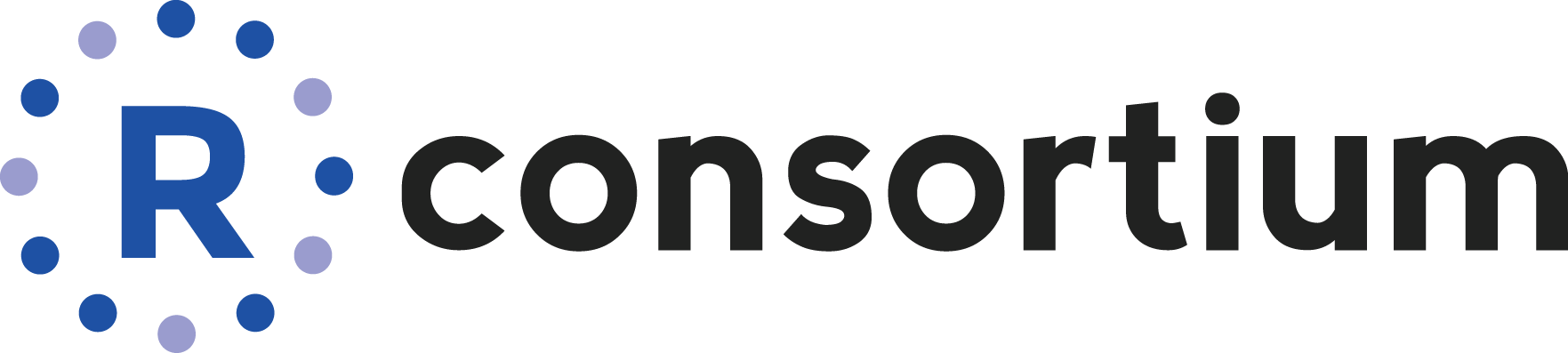# leafpm - Interactively Edit Spatial Vector Features in R

`leafpm` is a plugin for `leaflet` to provide map editing and drawing in R with `Leaflet.pm`. It is based closely off of `leaflet.extras` `addDrawToolbar()`. `leafpm` is intended to supplement `leaflet.extras` with better support for snapping and holes.

## Installation

You can install the released version of leafpm from CRAN with:

``install.packages("leafpm")``

## Example

``````library(mapview)
library(leafpm)
library(sf)

outer1 = matrix(c(0,0,10,0,10,10,0,10,0,0),ncol=2, byrow=TRUE)
hole1 = matrix(c(1,1,1,2,2,2,2,1,1,1),ncol=2, byrow=TRUE)
hole2 = matrix(c(5,5,5,6,6,6,6,5,5,5),ncol=2, byrow=TRUE)
outer2 = matrix(c(11,0,11,1,12,1,12,0,11,0),ncol=2, byrow=TRUE)

pts1 = list(outer1, hole1, hole2)
pts2 = list(outer2)

pl1 = st_sf(geom = st_sfc(st_polygon(pts1)))
pl2 = st_sf(geom = st_sfc(st_polygon(pts2)))

mpl = st_sf(geom = st_combine(rbind(pl1, pl2)))

mapview(mpl)@map,
targetGroup = "mpl"
)``````

## mapedit Integration

`leafpm` was designed to work as an editor in `mapedit`, so you can get your edits back into the `R` session. For instance,

``````#install.packages("mapedit")
library(mapedit)

drawFeatures(editor = "leafpm")``````

### Code of Conduct

Please note that the ‘leafpm’ project is released with a Contributor Code of Conduct. By participating in this project you agree to abide by its terms.

### Acknowledgment

This project has been realized with financial support from the